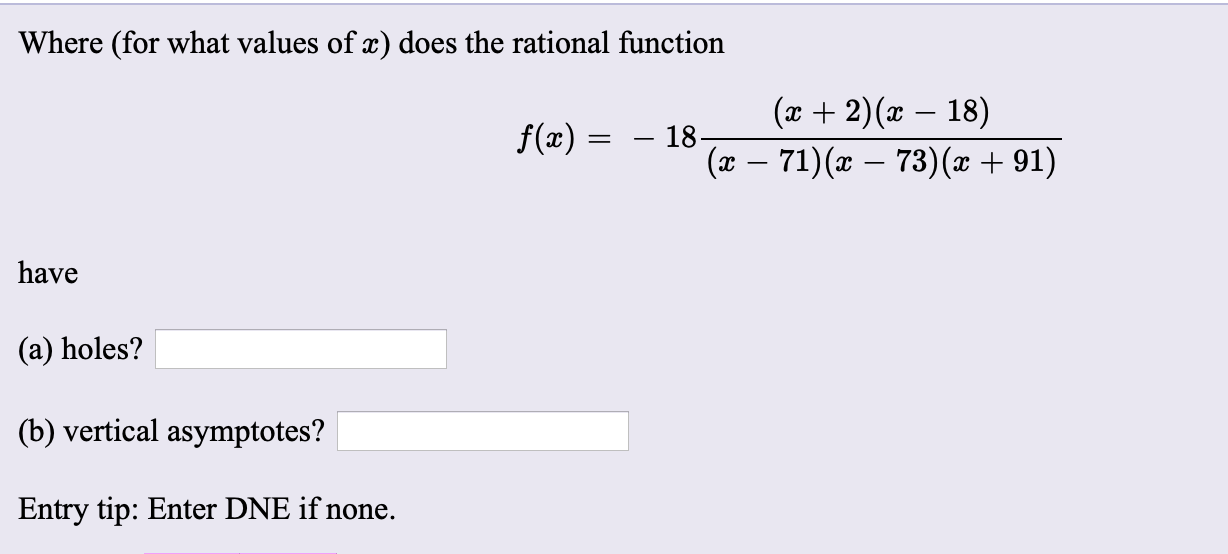# Where (for what values of x) does the rational function (x + 2)(x – 18) f(x) : – 18- (x – 71)(x – 73)(x + 91) have (a) holes? (b) vertical asymptotes? Entry tip: Enter DNE if none.

Question

please solve it and show yor workhelp_outlineImage TranscriptioncloseWhere (for what values of x) does the rational function (x + 2)(x – 18) f(x) : – 18- (x – 71)(x – 73)(x + 91) have (a) holes? (b) vertical asymptotes? Entry tip: Enter DNE if none. fullscreen